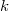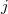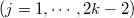﻿

### Polyharmonic maps into the Euclidean space

#### Abstract

We study polyharmonic ($k$-harmonic) maps between Riemannian manifolds with finite$j$-energies$(j=1,\cdots,2k-2)$. We show that if the domain is complete and the target is the Euclidean space, then such a map is harmonic.

DOI Code: 10.1285/i15900932v38n1p89

Keywords: harmonic map; polyharmonic map; Chen's conjecture; generalized Chen's conjecture

Full Text: PDF